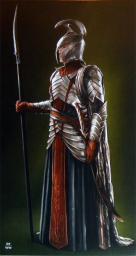# Contributors

Tolkien's languages appeals to a wide demography as the wide regional distribution of Parf Edhellen's visitors can attest. Our members come from all over the world, and they all have one thing in common: fascination with the beauty of the elvish languages.

On this page, we display top activity and contribution metrics by categories we believe you might be interested in. This page was generated and will be updated automatically .

## Most forum posts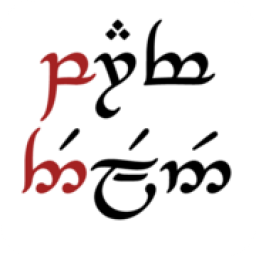406

12.54 % of total.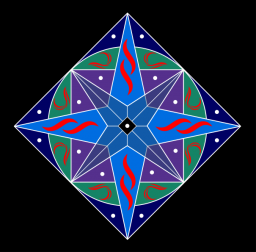220

6.79 % of total.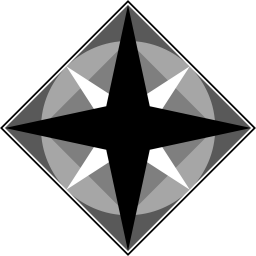195

6.02 % of total.143

4.42 % of total.121

3.74 % of total.

## Most flashcards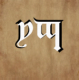6310

4.91 % of total.4855

3.78 % of total.4535

3.53 % of total.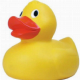4165

3.24 % of total.3953

3.08 % of total.

## Most phrases39

58.21 % of total.14

20.9 % of total.3

4.48 % of total.2

2.99 % of total.2

2.99 % of total.

## Most contributions53

20.95 % of total.17

6.72 % of total.16

6.32 % of total.15

5.93 % of total.14

5.53 % of total.143

14.39 % of total.131

13.18 % of total.99

9.96 % of total.87

8.75 % of total.62

6.24 % of total.

## Most glosses30803

65.44 % of total.5618

11.94 % of total.5495

11.67 % of total.4522

9.61 % of total.530

1.13 % of total.